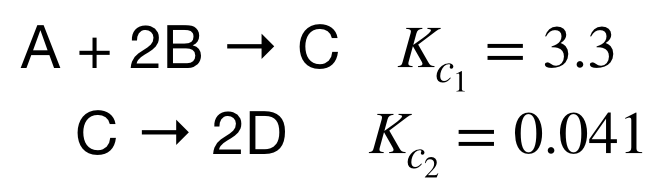# Problem: Calculate the value of the equilibrium constant K for the hypothetical reaction2D → A + 2Bfrom the following information:A + 2B → C   Kc = 3.3C → 2D       Kc = 0.041

###### FREE Expert Solution
96% (313 ratings)
###### FREE Expert Solution

Similar to Hess's Law, we must manipulate the given equations and Kc values to get the overall equation 2D → A + 2B.96% (313 ratings)###### Problem Details

Calculate the value of the equilibrium constant K for the hypothetical reaction

2D → A + 2B

from the following information:

A + 2B → C   K= 3.3

C → 2D       K= 0.041

What scientific concept do you need to know in order to solve this problem?

Our tutors have indicated that to solve this problem you will need to apply the Chemical Equilibrium concept. You can view video lessons to learn Chemical Equilibrium. Or if you need more Chemical Equilibrium practice, you can also practice Chemical Equilibrium practice problems.

What is the difficulty of this problem?

Our tutors rated the difficulty ofCalculate the value of the equilibrium constant K for the hy...as medium difficulty.

How long does this problem take to solve?

Our expert Chemistry tutor, Dasha took 3 minutes and 35 seconds to solve this problem. You can follow their steps in the video explanation above.

What professor is this problem relevant for?

Based on our data, we think this problem is relevant for Professor Neilson's class at CSU.